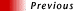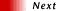| Browse the Best of RHF: "Computer, Science and Math Jokes" |# Math Purity Test

clubok%husc4@harvard.harvard.edu (Ken "The Snake" Clubok)
(science, original, chuckle)

This was made by a couple of friends of mine, Mike Bender and Sarah Herr:

MATHEMATICS PURITY TEST

Count the number of yeses, subtract from 60, and divide by 0.6.

#### The Basics

```1)  Have you ever been excited about math?
4)  Manipulated the numerator of an equation?
5)  Manipulated the denominator of an equation?
6)  On your first problem set?
7)  Worked on a problem set past 3:00 a.m.?
8)  Worked on a problem set all night?
10) Worked on a problem continuously for more than 30 minutes?
11) Worked on a problem continuously for more than four hours?
12) Done more than one problem set on the same night (i.e. both
started and finished them)?
13) Done more than three problem sets on the same night?
14) Taken a math course for a full year?
15) Taken two different math courses at the same time?
16) Done at least one problem set a week for more than four months?
17) Done at least one problem set a night for more than one month
(weekends excluded)?
18) Done a problem set alone?
19) Done a problem set in a group of three or more?
20) Done a problem set in a group of 15 or more?
21) Was it mixed company?
22) Have you ever inadvertently walked in upon people doing
a problem set?
23) And joined in afterwards?
24) Have you ever used food doing a problem set?
25) Did you eat it all?
26) Have you ever had a domesticated pet or animal walk over
you while you were doing a problem set?
27) Done a problem set in a public place where you might be discovered?
28) Been discovered while doing a problem set?

Kinky Stuff

29) Have you ever applied your math to a hard science?
30) Applied your math to a soft science?
31) Done an integration by parts?
32) Done two integration by parts in a single problem?
33) Bounded the domain and range of your function?
34) Used the domination test for improper integrals?
35) Done Newton's Method?
36) Done the Method of Frobenius?
37) Used the Sandwich Theorem?
38) Used the Mean Value Theorem?
39) Used a Gaussian surface?
40) Used a foreign object on a math problem (eg: calculator)?
41) Used a program to improve your mathematical technique (eg: MACSYMA)?
42) Not used brackets when you should have?
43) Integrated a function over its full period?
44) Done a calculation in three-dimensional space?
45) Done a calculation in n-dimensional space?
46) Done a change of bases?
47) Done a change of bases specifically in order to magnify your vector?
48) Worked through four complete bases in a single night (eg: using the
Gram-Schmidt method)?
49) Inserted a number into an equation?
50) Calculated the residue of a pole?
51) Scored perfectly on a math test?
52) Swallowed everything your professor gave you?
53) Used explicit notation in your problem set?
54) Purposefully omitted important steps in your problem set?| Browse the Best of RHF: "Computer, Science and Math Jokes" |